# Computational & Multiscale Mechanics of Materials

## Fracture Mechanics Online Class

Other online classes : Aircraft structures

# HRR theory > Other HRR solutions

In the previous section, we have derived the expressions of the near-tip stress and strain fields for a mode I crack in plane-$\varepsilon$ state. Moreover an uncompressible material has been assumed. In this section, a brief summary is given for other HRR solutions that were derived in the literature:

• Plane strain deformation near a crack tip in a power-law hardening material, J. R. Rice, G. F. Rosengren, Journal of Mechanics and Physics of Solids 16 (1968), 1-12;
• Singular behaviours at the end of a tensile crack in a hardening material, J. W. Hutchinson, Journal of Mechanics and Physics of Solids 16 (1968), 13-31;
• Plastic stress and strain fields at a crack tip, J. W. Hutchinson, Journal of Mechanics and Physics of Solids 16 (1968), 337-347;
• Fully plastic solutions and large scale yielding estimates for plane stress crack problems, C. F. Shih, J. W. Hutchinson, Journal of Engineering Materials and Technology 98 (1976), 289-295;
• Requirements for a one parameter characterization of crack tip fields by the HRR singularity, C. F. Shih, M. D. German, International Journal of Fracture 17(1) (1981), 27- 43.

## Mode I crack in plane-$\sigma$ state

Still assuming an uncompressible material, a similar analysis as in the plane-$\varepsilon$ state can be performed and yields different components tensors $\tilde{\cauchy} \left(\theta,n\right)$, $\tilde{\strain} \left(\theta,n\right)$, and $\tilde{\bf{u}} \left(\theta,n\right)$ fields.

### Near-tip stress field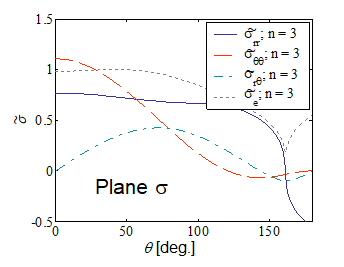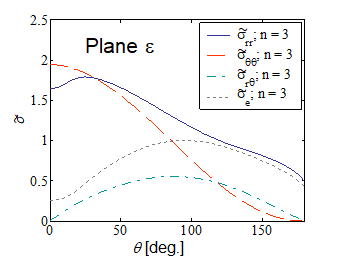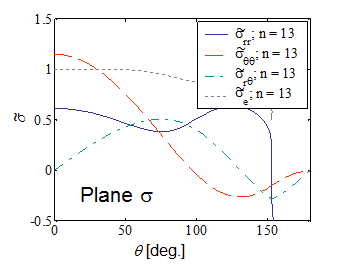As we have derived in the previous section, the near-tip stress field reads

\begin{equation} \cauchy=\sigma_p^0\left(\frac{JE}{r\alpha\left(\sigma_p^0\right)^2I_n}\right)^{\frac{1}{n+1}}\tilde{\cauchy}\left(\theta,\,n\right) \, \text{.} \end{equation}.

Pictures VIII.17-VIII.20 compare the stress shape tensor $\tilde{\sigma}$ components between plane-$\sigma$ and plane-$\varepsilon$ conditions for two different hardening parameters. Moreover the integral $I_n$ is reported in Picture VIII.21 for the two different cases. These figures allow comparing the difference in the crack tip loading, for instance through the asymptotic hoop stress $\sigma_{\theta\theta}$, between a plane-$\varepsilon$ and a plane-$\sigma$ states. Indeed, for the same value of the $J$-integral, and at the same crack tip distance $r$, the ratio between the two hoop stresses for two different hardening parameters are

\begin{equation} \frac{\left.\cauchy_{\theta\theta}\left(0,\,r\right)\right|_{n=3,\,\text{plane }-\varepsilon}}{\left.\cauchy_{\theta\theta}\left(0,\,r\right)\right|_{n=3,\,\text{plane}-\sigma}} \simeq\frac{1.9}{1.1}\left(\frac{3.86}{5.81}\right)^\frac{1}{4} = 1.56 \, \text{,} \end{equation} \begin{equation} \frac{\left.\cauchy_{\theta\theta}\left(0,\,r\right)\right|_{n=13,\,\text{plane}-varepsilon}}{\left.\cauchy_{\theta\theta}\left(0,\,r\right)\right|_{n=13,\,\text{plane}-\sigma}} \simeq\frac{2.6}{1.2}\left(\frac{2.87}{3.4}\right)^\frac{1}{14} = 2.13\,. \end{equation}

It can be seen that because the material cannot expend laterally, the crack tip is more loading in plane-$\varepsilon$ state, for the same value of the $J$-integral.

### Process zone shapes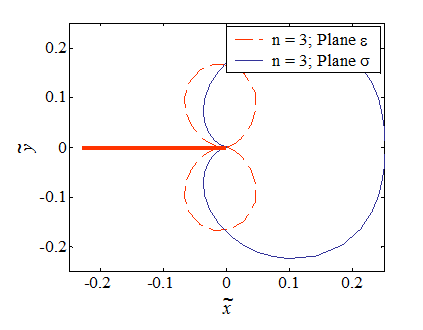The process zone was defined as the zone in which $\sigma_e > \sigma_p^0$, whose boundary corresponds to $\sigma_e=\sigma_p^0$, and was found to be

\begin{equation} \tilde{r}\left(\theta,\,n\right) = \frac{\left(\tilde{\sigma}_e\left(\theta,\,n\right)\right)^{n+1}}{I_n} \, \text{.}\label{eq:processZone} \end{equation}

in terms of a non-dimensional radius $\tilde{r}= \frac{r \alpha \left(\sigma_p^0\right)^2 }{JE}$. The process zones for $n=$ are illustrated in Picture VIII.24 for the plane-$\sigma$ and plane-$\varepsilon$ states. It can be seen that the process zone is more diffuse in plane-$\sigma$ state.

### Crack Tip Opening Displacement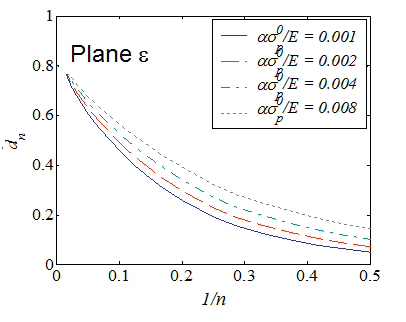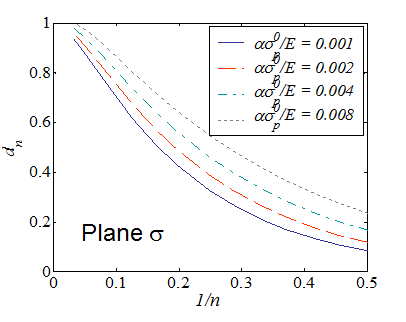We have seen that there exists a direct relation between the crack tip opening displacement and the $J$-integral:

\begin{equation} \delta_t = d_n \frac{J}{\sigma^0_p}\,, \end{equation}

where $d_n$ depends on $n$ but also on the elastic strain of the considered material $\frac{\alpha\sigma^0_p}{E}$. The evolutions of $d_n$ in the cases of plane-$\varepsilon$ and plane-$\sigma$ states are respectively illustrated in Picture VIII.22 and Picture VIII.23.Assuming SSY, the process zone develops under mode III following a circular shape, as illustrated in Picture VIII.25 and Picture VIII.26 for respectively a perfectly plastic material and an elasto-plastic material with strain hardening.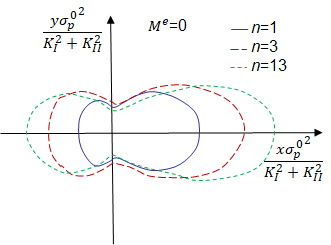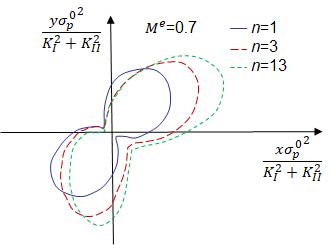In SSY, the solution depends on the elastic mixity parameter given by

\begin{equation} M^e = \frac{2}{\pi}\arctan{\frac{K_I}{K_{II}}}\,, \end{equation}

which ranges from 0 (Mode I crack loading) to 1 (Mode II crack loading). Varying the mixity parameter the process zone changes orientation, from a"horizontal" shape in Mode II crack loading, as depicted in Picture VIII.27, rotating upward for a mixed mode crack loading as depicted in Picture VIII.28.

## Compressibility effect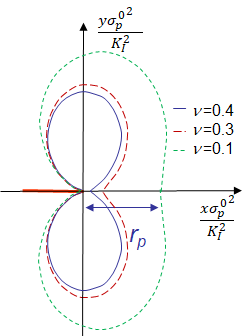The HRR theory was obtained assuming an incompressible material. Using the finite element approach, it is possible to solve the problem with a real elasto-plastic law for which the elastic part follows a classical Hooke's law characterized by a Poisson ratio $\nu$. Considering a crack loaded in mode I under plane-$\varepsilon$ state and assuming SSY, the effect of the Poisson ratio on the process zone is depicted in Picture VIII.29. The plastic zone size $r_p$ is defined as the length of the plastic zone ahead of the crack tip, and increases with the material compressibility.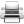Last update: September 02, 2015